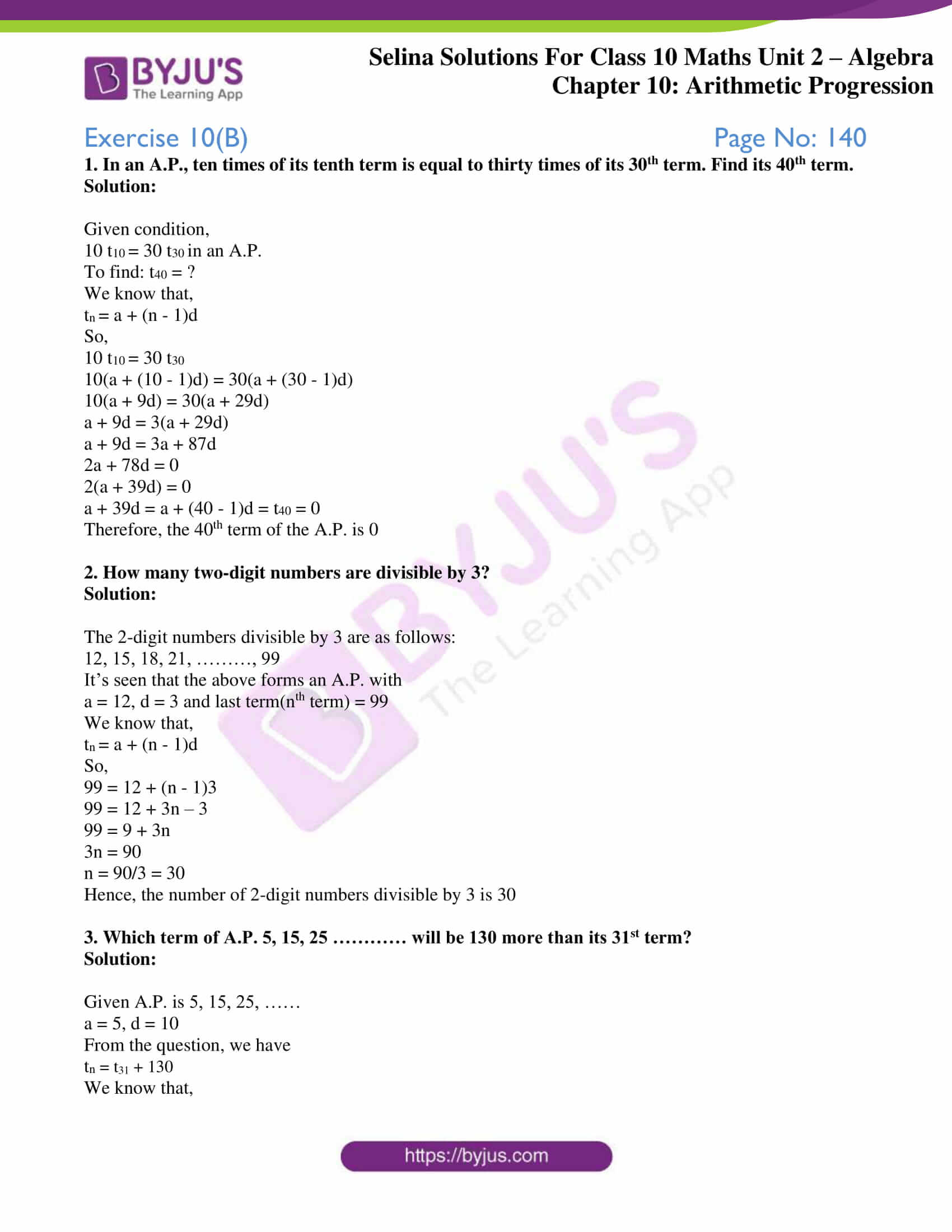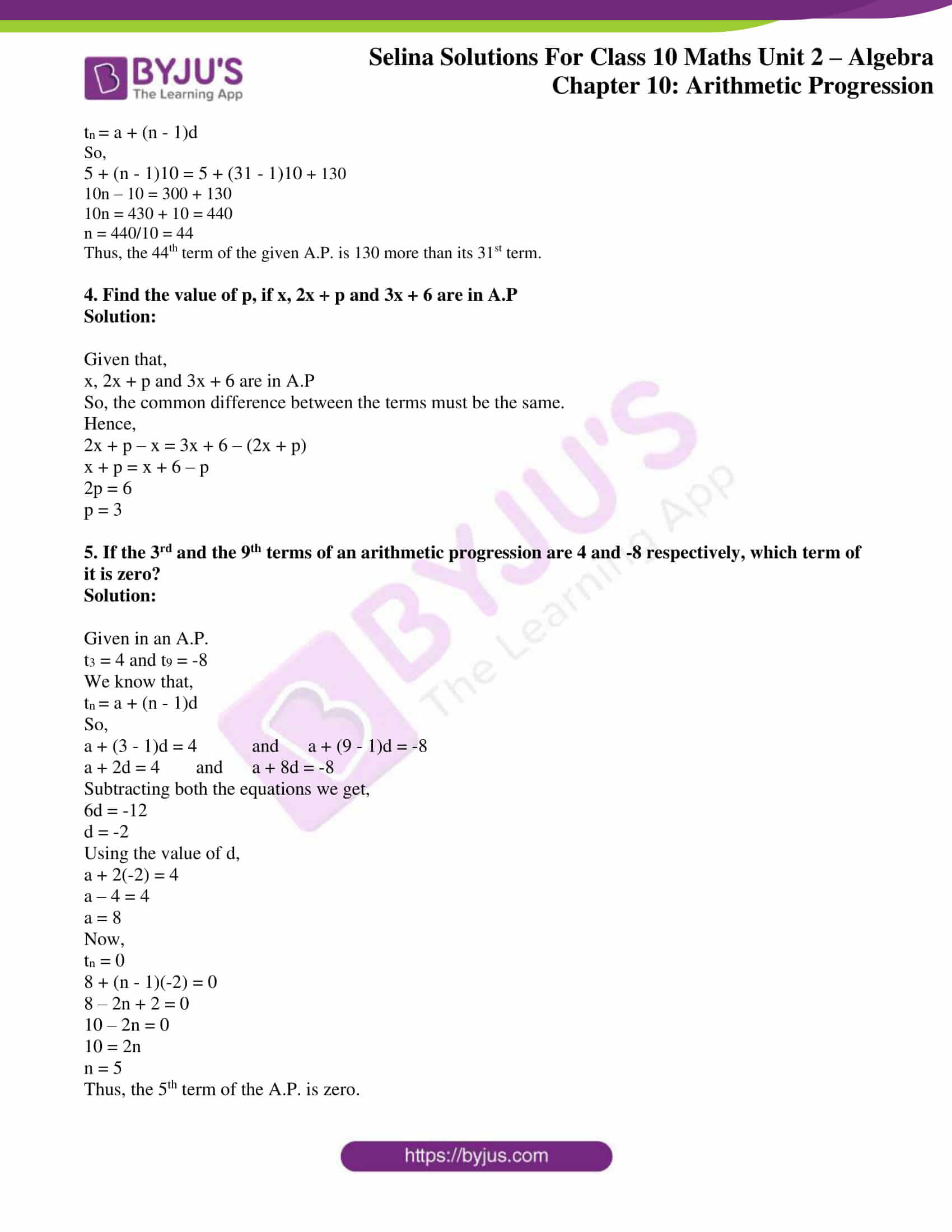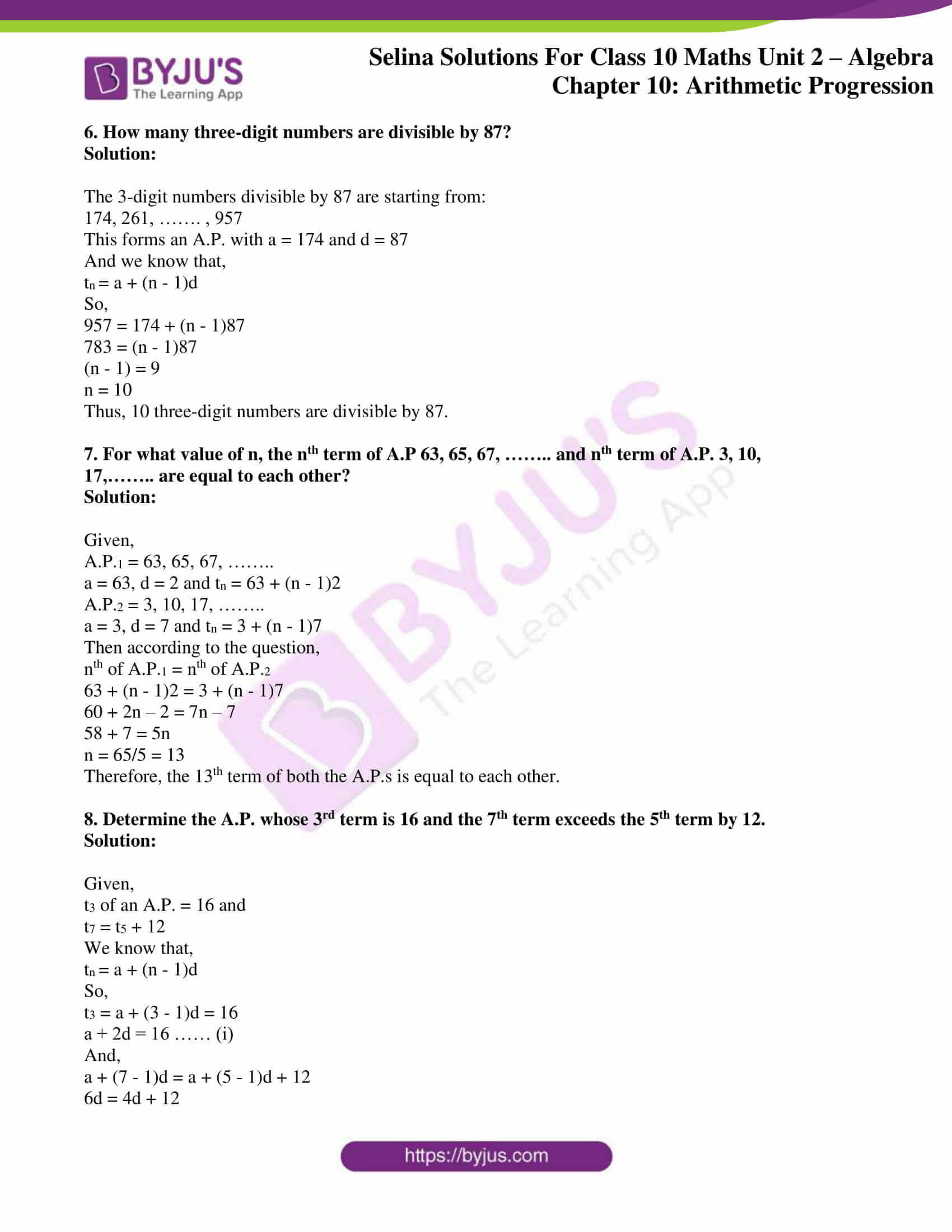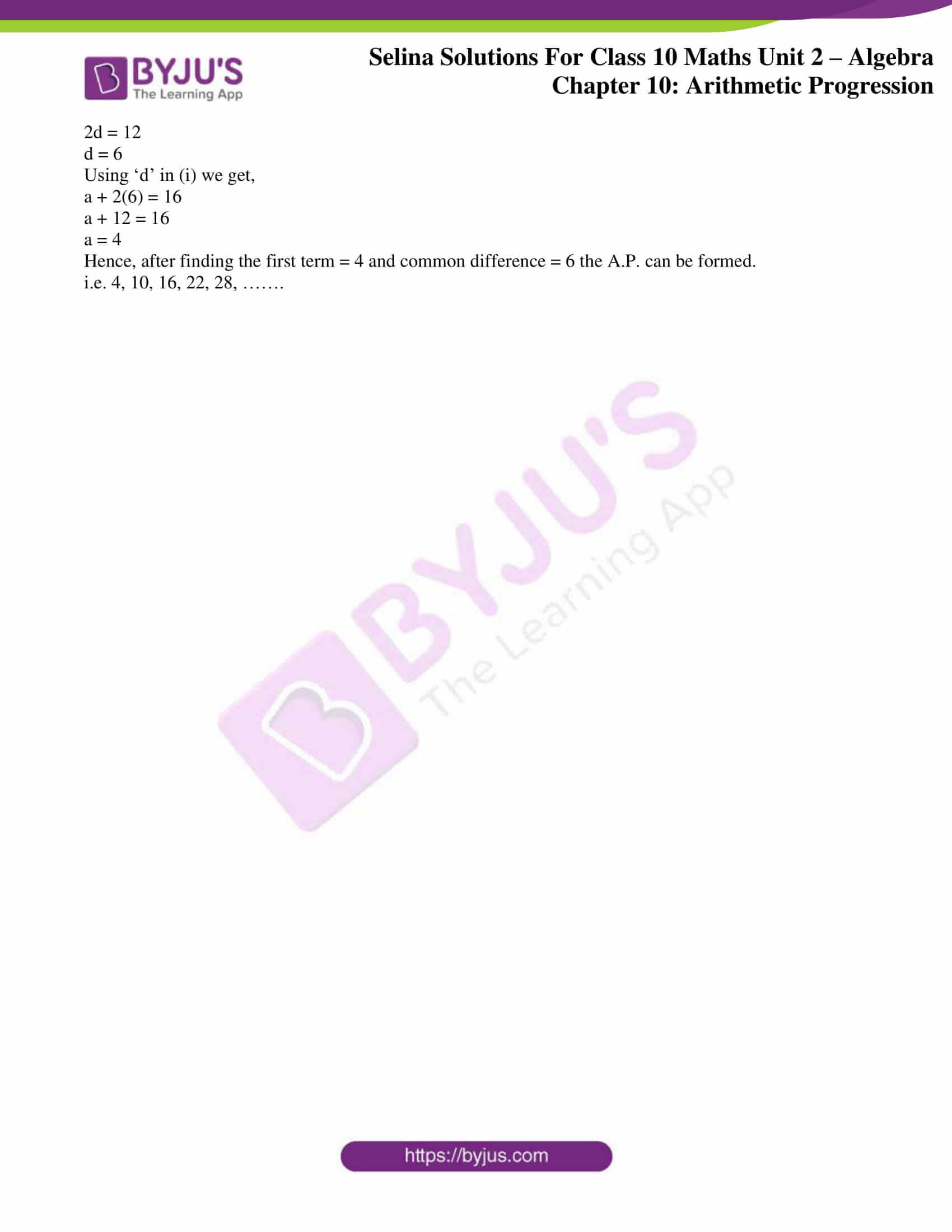# Selina Solutions Concise Maths Class 10 Chapter 10 Arithmetic Progression Exercise 10(B)

More problems on finding the terms of an A.P. when given certain conditions are included in this exercise. Students who want to learn the correct steps of solving problems of this exercise and chapter can make use of the Selina Solutions for Class 10 Maths PDF. For accurate answers of this exercise problems, the Selina Solutions Concise Maths Class 10 Chapter 10 Arithmetic Progression Exercise 10(B) PDF is available for reference in the links given below.

## Selina Solutions Concise Maths Class 10 Chapter 10 Arithmetic Progression Exercise 10(B) Download PDF### Access Selina Solutions Concise Maths Class 10 Chapter 10 Arithmetic Progression Exercise 10(B)

#### Exercise 10(B) Page No: 140

1. In an A.P., ten times of its tenth term is equal to thirty times of its 30th term. Find its 40th term.

Solution:

Given condition,

10 t10 = 30 t30 in an A.P.

To find: t40 = ?

We know that,

tn = a + (n – 1)d

So,

10 t10 = 30 t30

10(a + (10 – 1)d) = 30(a + (30 – 1)d)

10(a + 9d) = 30(a + 29d)

a + 9d = 3(a + 29d)

a + 9d = 3a + 87d

2a + 78d = 0

2(a + 39d) = 0

a + 39d = a + (40 – 1)d = t40 = 0

Therefore, the 40th term of the A.P. is 0

2. How many two-digit numbers are divisible by 3?

Solution:

The 2-digit numbers divisible by 3 are as follows:

12, 15, 18, 21, ………, 99

It’s seen that the above forms an A.P. with

a = 12, d = 3 and last term(nth term) = 99

We know that,

tn = a + (n – 1)d

So,

99 = 12 + (n – 1)3

99 = 12 + 3n – 3

99 = 9 + 3n

3n = 90

n = 90/3 = 30

Hence, the number of 2-digit numbers divisible by 3 is 30

3. Which term of A.P. 5, 15, 25 ………… will be 130 more than its 31st term?

Solution:

Given A.P. is 5, 15, 25, ……

a = 5, d = 10

From the question, we have

tn = t31 + 130

We know that,

tn = a + (n – 1)d

So,

5 + (n – 1)10 = 5 + (31 – 1)10 + 130

10n – 10 = 300 + 130

10n = 430 + 10 = 440

n = 440/10 = 44

Thus, the 44th term of the given A.P. is 130 more than its 31st term.

4. Find the value of p, if x, 2x + p and 3x + 6 are in A.P

Solution:

Given that,

x, 2x + p and 3x + 6 are in A.P

So, the common difference between the terms must be the same.

Hence,

2x + p – x = 3x + 6 – (2x + p)

x + p = x + 6 – p

2p = 6

p = 3

5. If the 3rd and the 9th terms of an arithmetic progression are 4 and -8 respectively, which term of it is zero?

Solution:

Given in an A.P.

t3 = 4 and t9 = -8

We know that,

tn = a + (n – 1)d

So,

a + (3 – 1)d = 4 and a + (9 – 1)d = -8

a + 2d = 4 and a + 8d = -8

Subtracting both the equations we get,

6d = -12

d = -2

Using the value of d,

a + 2(-2) = 4

a – 4 = 4

a = 8

Now,

tn = 0

8 + (n – 1)(-2) = 0

8 – 2n + 2 = 0

10 – 2n = 0

10 = 2n

n = 5

Thus, the 5th term of the A.P. is zero.

6. How many three-digit numbers are divisible by 87?

Solution:

The 3-digit numbers divisible by 87 are starting from:

174, 261, ……. , 957

This forms an A.P. with a = 174 and d = 87

And we know that,

tn = a + (n – 1)d

So,

957 = 174 + (n – 1)87

783 = (n – 1)87

(n – 1) = 9

n = 10

Thus, 10 three-digit numbers are divisible by 87.

7. For what value of n, the nth term of A.P 63, 65, 67, …….. and nth term of A.P. 3, 10, 17,…….. are equal to each other?

Solution:

Given,

A.P.1 = 63, 65, 67, ……..

a = 63, d = 2 and tn = 63 + (n – 1)2

A.P.2 = 3, 10, 17, ……..

a = 3, d = 7 and tn = 3 + (n – 1)7

Then according to the question,

nth of A.P.1 = nth of A.P.2

63 + (n – 1)2 = 3 + (n – 1)7

60 + 2n – 2 = 7n – 7

58 + 7 = 5n

n = 65/5 = 13

Therefore, the 13th term of both the A.P.s is equal to each other.

8. Determine the A.P. whose 3rd term is 16 and the 7th term exceeds the 5th term by 12.

Solution:

Given,

t3 of an A.P. = 16 and

t7 = t5 + 12

We know that,

tn = a + (n – 1)d

So,

t3 = a + (3 – 1)d = 16

a + 2d = 16 …… (i)

And,

a + (7 – 1)d = a + (5 – 1)d + 12

6d = 4d + 12

2d = 12

d = 6

Using ‘d’ in (i) we get,

a + 2(6) = 16

a + 12 = 16

a = 4

Hence, after finding the first term = 4 and common difference = 6 the A.P. can be formed.

i.e. 4, 10, 16, 22, 28, …….

### Access other exercises of Selina Solutions Concise Maths Class 10 Chapter 10 Arithmetic Progression

Exercise 10(A) Solutions

Exercise 10(C) Solutions

Exercise 10(D) Solutions

Exercise 10(E) Solutions

Exercise 10(F) Solutions